# 4 编写结构化程序

1. 怎么能写出结构良好、可读的程序，你和其他人将能够很容易的重新使用它？
2. 基本结构块，如循环、函数以及赋值，是如何执行的？
3. Python 编程的陷阱有哪些，你怎么能避免它们吗？

## 4.1 回到基础

### 赋值

```>>> foo = 'Monty'
>>> bar = foo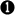>>> foo = 'Python'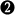>>> bar
'Monty'```

```>>> foo = ['Monty', 'Python']
>>> bar = foo>>> foo = 'Bodkin'>>> bar
['Monty', 'Bodkin']```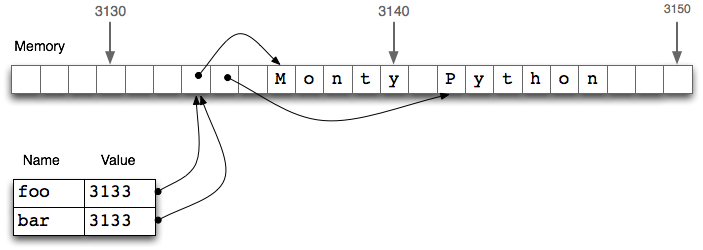bar = foo行并不会复制变量的内容，只有它的“引用对象”。要了解这里发生了什么事，我们需要知道列表是如何存储在计算机内存的。4.1中，我们看到一个列表foo是对存储在位置3133处的一个对象的引用（它自身是一个指针序列，其中的指针指向其它保存字符串的位置）。当我们赋值bar = foo时，仅仅是3133位置处的引用被复制。这种行为延伸到语言的其他方面，如参数传递（4.4）。

```>>> empty = []
>>> nested = [empty, empty, empty]
>>> nested
[[], [], []]
>>> nested.append('Python')
>>> nested
[['Python'], ['Python'], ['Python']]```

```>>> nested = [[]] * 3
>>> nested.append('Python')
>>> nested = ['Monty']
>>> nested
[['Python'], ['Monty'], ['Python']]```

### 等式

Python提供两种方法来检查一对项目是否相同。is操作符测试对象的ID。我们可以用它来验证我们早先的对对象的观察。首先，我们创建一个列表，其中包含同一对象的多个副本，证明它们不仅对于==完全相同，而且它们是同一个对象：

```>>> size = 5
>>> python = ['Python']
>>> snake_nest = [python] * size
>>> snake_nest == snake_nest == snake_nest == snake_nest == snake_nest
True
>>> snake_nest is snake_nest is snake_nest is snake_nest is snake_nest
True```

```>>> import random
>>> position = random.choice(range(size))
>>> snake_nest[position] = ['Python']
>>> snake_nest
[['Python'], ['Python'], ['Python'], ['Python'], ['Python']]
>>> snake_nest == snake_nest == snake_nest == snake_nest == snake_nest
True
>>> snake_nest is snake_nest is snake_nest is snake_nest is snake_nest
False```

```>>> [id(snake) for snake in snake_nest]
[4557855488, 4557854763, 4557855488, 4557855488, 4557855488]```

### 条件

if语句的条件部分，一个非空字符串或列表被求值为真，而一个空字符串或列表的被求值为假。

```>>> mixed = ['cat', '', ['dog'], []]
>>> for element in mixed:
...     if element:
...         print(element)
...
cat
['dog']```

```>>> animals = ['cat', 'dog']
>>> if 'cat' in animals:
...     print(1)
... elif 'dog' in animals:
...     print(2)
...
1```

all()函数和any()函数可以应用到一个列表（或其他序列），来检查是否全部或任一项目满足某个条件：

```>>> sent = ['No', 'good', 'fish', 'goes', 'anywhere', 'without', 'a', 'porpoise', '.']
>>> all(len(w) > 4 for w in sent)
False
>>> any(len(w) > 4 for w in sent)
True```

## 4.2 序列

```>>> t = 'walk', 'fem', 3>>> t
('walk', 'fem', 3)
>>> t'walk'
>>> t[1:]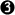('fem', 3)
>>> len(t)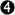3```

```>>> raw = 'I turned off the spectroroute'
>>> text = ['I', 'turned', 'off', 'the', 'spectroroute']
>>> pair = (6, 'turned')
>>> raw, text, pair
('t', 'the', 'turned')
>>> raw[-3:], text[-3:], pair[-3:]
('ute', ['off', 'the', 'spectroroute'], (6, 'turned'))
>>> len(raw), len(text), len(pair)
(29, 5, 2)```

### 序列类型上的操作

```>>> raw = 'Red lorry, yellow lorry, red lorry, yellow lorry.'
>>> text = word_tokenize(raw)
>>> fdist = nltk.FreqDist(text)
>>> sorted(fdist)
[',', '.', 'Red', 'lorry', 'red', 'yellow']
>>> for key in fdist:
...     print(key + ':', fdist[key], end='; ')
...
lorry: 4; red: 1; .: 1; ,: 3; Red: 1; yellow: 2```

```>>> words = ['I', 'turned', 'off', 'the', 'spectroroute']
>>> words, words, words = words, words, words
>>> words
['I', 'turned', 'the', 'spectroroute', 'off']```

```>>> tmp = words
>>> words = words
>>> words = words
>>> words = tmp```

```>>> words = ['I', 'turned', 'off', 'the', 'spectroroute']
>>> tags = ['noun', 'verb', 'prep', 'det', 'noun']
>>> zip(words, tags)
<zip object at ...>
>>> list(zip(words, tags))
[('I', 'noun'), ('turned', 'verb'), ('off', 'prep'),
('the', 'det'), ('spectroroute', 'noun')]
>>> list(enumerate(words))
[(0, 'I'), (1, 'turned'), (2, 'off'), (3, 'the'), (4, 'spectroroute')]```

```>>> text = nltk.corpus.nps_chat.words()
>>> cut = int(0.9 * len(text))>>> training_data, test_data = text[:cut], text[cut:]>>> text == training_data + test_dataTrue
>>> len(training_data) / len(test_data)9.0```

### 合并不同类型的序列

```>>> words = 'I turned off the spectroroute'.split()>>> wordlens = [(len(word), word) for word in words]>>> wordlens.sort()>>> ' '.join(w for (_, w) in wordlens)'I off the turned spectroroute'```

```>>> lexicon = [
...     ('the', 'det', ['Di:', 'D@']),
...     ('off', 'prep', ['Qf', 'O:f'])
... ]```

```>>> lexicon.sort()
>>> lexicon = ('turned', 'VBD', ['t3:nd', 't3`nd'])
>>> del lexicon```

### 生成器表达式

```>>> text = '''"When I use a word," Humpty Dumpty said in rather a scornful tone,
... "it means just what I choose it to mean - neither more nor less."'''
>>> [w.lower() for w in word_tokenize(text)]
['``', 'when', 'i', 'use', 'a', 'word', ',', "''", 'humpty', 'dumpty', 'said', ...]```

```>>> max([w.lower() for w in word_tokenize(text)])'word'
>>> max(w.lower() for w in word_tokenize(text))'word'```

## 4.3 风格的问题

### Python代码风格

```>>> if (len(syllables) > 4 and len(syllables) == 3 and
...    syllables in [aeiou] and syllables == syllables):
...     process(syllables)
>>> if len(syllables) > 4 and len(syllables) == 3 and \
...    syllables in [aeiou] and syllables == syllables:
...     process(syllables)```

### 过程风格与声明风格

```>>> tokens = nltk.corpus.brown.words(categories='news')
>>> count = 0
>>> total = 0
>>> for token in tokens:
...     count += 1
...     total += len(token)
>>> total / count
4.401545438271973```

```>>> total = sum(len(t) for t in tokens)
>>> print(total / len(tokens))
4.401...```

```>>> word_list = []
>>> i = 0
>>> while i < len(tokens):
...     j = 0
...     while j < len(word_list) and word_list[j] <= tokens[i]:
...         j += 1
...     if j == 0 or tokens[i] != word_list[j-1]:
...         word_list.insert(j, tokens[i])
...     i += 1
...```

`>>> word_list = sorted(set(tokens))`

```>>> fd = nltk.FreqDist(nltk.corpus.brown.words())
>>> cumulative = 0.0
>>> most_common_words = [word for (word, count) in fd.most_common()]
>>> for rank, word in enumerate(most_common_words):
...     cumulative += fd.freq(word)
...     print("%3d %6.2f%% %s" % (rank + 1, cumulative * 100, word))
...     if cumulative > 0.25:
...         break
...
1   5.40% the
2  10.42% ,
3  14.67% .
4  17.78% of
5  20.19% and
6  22.40% to
7  24.29% a
8  25.97% in```

```>>> text = nltk.corpus.gutenberg.words('milton-paradise.txt')
>>> longest = ''
>>> for word in text:
...     if len(word) > len(longest):
...         longest = word
>>> longest
'unextinguishable'```

```>>> maxlen = max(len(word) for word in text)
>>> [word for word in text if len(word) == maxlen]
['unextinguishable', 'transubstantiate', 'inextinguishable', 'incomprehensible']```

### 计数器的一些合理用途

```>>> sent = ['The', 'dog', 'gave', 'John', 'the', 'newspaper']
>>> n = 3
>>> [sent[i:i+n] for i in range(len(sent)-n+1)]
[['The', 'dog', 'gave'],
['dog', 'gave', 'John'],
['gave', 'John', 'the'],
['John', 'the', 'newspaper']]```

```>>> m, n = 3, 7
>>> array = [[set() for i in range(n)] for j in range(m)]
>>> array.add('Alice')
>>> pprint.pprint(array)
[[set(), set(), set(), set(), set(), set(), set()],
[set(), set(), set(), set(), set(), set(), set()],
[set(), set(), set(), set(), set(), {'Alice'}, set()]]```

```>>> array = [[set()] * n] * m
>>> array.add(7)
>>> pprint.pprint(array)
[[{7}, {7}, {7}, {7}, {7}, {7}, {7}],
[{7}, {7}, {7}, {7}, {7}, {7}, {7}],
[{7}, {7}, {7}, {7}, {7}, {7}, {7}]]```

## 4.4 函数：结构化编程的基础

```import re
def get_text(file):
"""Read text from a file, normalizing whitespace and stripping HTML markup."""
text = open(file).read()
text = re.sub(r'<.*?>', ' ', text)
text = re.sub('\s+', ' ', text)
return text```

```|   >>> help(get_text)
|   Help on function get_text in module __main__:
|
|   get(text)
|       Read text from a file, normalizing whitespace and stripping HTML markup.
```

```>>> def monty():
...     return "Monty Python"
>>> monty()
'Monty Python'```

```>>> repeat(monty(), 3)
'Monty Python Monty Python Monty Python'
>>> repeat('Monty Python', 3)
'Monty Python Monty Python Monty Python'```

```>>> def my_sort1(mylist):      # good: modifies its argument, no return value
...     mylist.sort()
>>> def my_sort2(mylist):      # good: doesn't touch its argument, returns value
...     return sorted(mylist)
>>> def my_sort3(mylist):      # bad: modifies its argument and also returns it
...     mylist.sort()
...     return mylist```

### 参数传递

```>>> def set_up(word, properties):
...     word = 'lolcat'
...     properties.append('noun')
...     properties = 5
...
>>> w = ''
>>> p = []
>>> set_up(w, p)
>>> w
''
>>> p
['noun']```

```>>> w = ''
>>> word = w
>>> word = 'lolcat'
>>> w
''```

```>>> p = []
>>> properties = p
>>> properties.append('noun')
>>> properties = 5
>>> p
['noun']```

### 参数类型检查

```>>> def tag(word):
...     if word in ['a', 'the', 'all']:
...         return 'det'
...     else:
...         return 'noun'
...
>>> tag('the')
'det'
>>> tag('knight')
'noun'
>>> tag(["'Tis", 'but', 'a', 'scratch'])'noun'```

```>>> def tag(word):
...     assert isinstance(word, basestring), "argument to tag() must be a string"
...     if word in ['a', 'the', 'all']:
...         return 'det'
...     else:
...         return 'noun'```

### 功能分解

```>>> data = load_corpus()
>>> results = analyze(data)
>>> present(results)```

```from urllib import request
from bs4 import BeautifulSoup

def freq_words(url, freqdist, n):
html = request.urlopen(url).read().decode('utf8')
raw = BeautifulSoup(html).get_text()
for word in word_tokenize(raw):
freqdist[word.lower()] += 1
result = []
for word, count in freqdist.most_common(n):
result = result + [word]
print(result)```

```from urllib import request
from bs4 import BeautifulSoup

def freq_words(url, n):
html = request.urlopen(url).read().decode('utf8')
text = BeautifulSoup(html).get_text()
freqdist = nltk.FreqDist(word.lower() for word in word_tokenize(text))
return [word for (word, _) in fd.most_common(n)]```

freq_words函数的可读性和可用性得到改进。

### 编写函数的文档

```def accuracy(reference, test):
"""
Calculate the fraction of test items that equal the corresponding reference items.

Given a list of reference values and a corresponding list of test values,
return the fraction of corresponding values that are equal.
In particular, return the fraction of indexes
{0<i<=len(test)} such that C{test[i] == reference[i]}.

>>> accuracy(['ADJ', 'N', 'V', 'N'], ['N', 'N', 'V', 'ADJ'])
0.5

:param reference: An ordered list of reference values
:type reference: list
:param test: A list of values to compare against the corresponding
reference values
:type test: list
:return: the accuracy score
:rtype: float
:raises ValueError: If reference and length do not have the same length
"""

if len(reference) != len(test):
raise ValueError("Lists must have the same length.")
num_correct = 0
for x, y in zip(reference, test):
if x == y:
num_correct += 1
return float(num_correct) / len(reference)```

## 4.5 更多关于函数

### 作为参数的函数

```>>> sent = ['Take', 'care', 'of', 'the', 'sense', ',', 'and', 'the',
...         'sounds', 'will', 'take', 'care', 'of', 'themselves', '.']
>>> def extract_property(prop):
...     return [prop(word) for word in sent]
...
>>> extract_property(len)
[4, 4, 2, 3, 5, 1, 3, 3, 6, 4, 4, 4, 2, 10, 1]
>>> def last_letter(word):
...     return word[-1]
>>> extract_property(last_letter)
['e', 'e', 'f', 'e', 'e', ',', 'd', 'e', 's', 'l', 'e', 'e', 'f', 's', '.']```

Python提供了更多的方式来定义函数作为其他函数的参数，即所谓的lambda 表达式试想在很多地方没有必要使用上述的last_letter()函数，因此没有必要给它一个名字。我们可以等价地写以下内容：

```>>> extract_property(lambda w: w[-1])
['e', 'e', 'f', 'e', 'e', ',', 'd', 'e', 's', 'l', 'e', 'e', 'f', 's', '.']```

```>>> sorted(sent)
[',', '.', 'Take', 'and', 'care', 'care', 'of', 'of', 'sense', 'sounds',
'take', 'the', 'the', 'themselves', 'will']
>>> sorted(sent, cmp)
[',', '.', 'Take', 'and', 'care', 'care', 'of', 'of', 'sense', 'sounds',
'take', 'the', 'the', 'themselves', 'will']
>>> sorted(sent, lambda x, y: cmp(len(y), len(x)))
['themselves', 'sounds', 'sense', 'Take', 'care', 'will', 'take', 'care',
'the', 'and', 'the', 'of', 'of', ',', '.']```

### 累计函数

```def search1(substring, words):
result = []
for word in words:
if substring in word:
result.append(word)
return result

def search2(substring, words):
for word in words:
if substring in word:
yield word```

```>>> def permutations(seq):
...     if len(seq) <= 1:
...         yield seq
...     else:
...         for perm in permutations(seq[1:]):
...             for i in range(len(perm)+1):
...                 yield perm[:i] + seq[0:1] + perm[i:]
...
>>> list(permutations(['police', 'fish', 'buffalo']))[['police', 'fish', 'buffalo'], ['fish', 'police', 'buffalo'],
['fish', 'buffalo', 'police'], ['police', 'buffalo', 'fish'],
['buffalo', 'police', 'fish'], ['buffalo', 'fish', 'police']]```

permutations函数使用了一种技术叫递归，将在下面4.7讨论。产生一组词的排列对于创建测试一个语法的数据十分有用（8.)。

### 高阶函数

Python提供一些具有函数式编程语言如Haskell标准特征的高阶函数。我们将在这里演示它们，与使用列表推导的相对应的表达一起。

```>>> def is_content_word(word):
...     return word.lower() not in ['a', 'of', 'the', 'and', 'will', ',', '.']
>>> sent = ['Take', 'care', 'of', 'the', 'sense', ',', 'and', 'the',
...         'sounds', 'will', 'take', 'care', 'of', 'themselves', '.']
>>> list(filter(is_content_word, sent))
['Take', 'care', 'sense', 'sounds', 'take', 'care', 'themselves']
>>> [w for w in sent if is_content_word(w)]
['Take', 'care', 'sense', 'sounds', 'take', 'care', 'themselves']```

```>>> lengths = list(map(len, nltk.corpus.brown.sents(categories='news')))
>>> sum(lengths) / len(lengths)
21.75081116158339
>>> lengths = [len(sent) for sent in nltk.corpus.brown.sents(categories='news')]
>>> sum(lengths) / len(lengths)
21.75081116158339```

```>>> list(map(lambda w: len(filter(lambda c: c.lower() in "aeiou", w)), sent))
[2, 2, 1, 1, 2, 0, 1, 1, 2, 1, 2, 2, 1, 3, 0]
>>> [len(c for c in w if c.lower() in "aeiou") for w in sent]
[2, 2, 1, 1, 2, 0, 1, 1, 2, 1, 2, 2, 1, 3, 0]```

### 命名的参数

```>>> def repeat(msg='<empty>', num=1):
...     return msg * num
>>> repeat(num=3)
'<empty><empty><empty>'
>>> repeat(msg='Alice')
'Alice'
>>> repeat(num=5, msg='Alice')
'AliceAliceAliceAliceAlice'```

```>>> def generic(*args, **kwargs):
...     print(args)
...     print(kwargs)
...
>>> generic(1, "African swallow", monty="python")
(1, 'African swallow')
{'monty': 'python'}```

*args作为函数参数时，它实际上对应函数所有的未命名参数。下面是另一个这方面的Python语法的演示，处理可变数目的参数的函数zip()我们将使用变量名*song来表示名字*args并没有什么特别的。

```>>> song = [['four', 'calling', 'birds'],
...         ['three', 'French', 'hens'],
...         ['two', 'turtle', 'doves']]
>>> list(zip(song, song, song))
[('four', 'three', 'two'), ('calling', 'French', 'turtle'), ('birds', 'hens', 'doves')]
>>> list(zip(*song))
[('four', 'three', 'two'), ('calling', 'French', 'turtle'), ('birds', 'hens', 'doves')]```

```>>> def freq_words(file, min=1, num=10):
...     text = open(file).read()
...     tokens = word_tokenize(text)
...     freqdist = nltk.FreqDist(t for t in tokens if len(t) >= min)
...     return freqdist.most_common(num)
>>> fw = freq_words('ch01.rst', 4, 10)
>>> fw = freq_words('ch01.rst', min=4, num=10)
>>> fw = freq_words('ch01.rst', num=10, min=4)```

```>>> def freq_words(file, min=1, num=10, verbose=False):
...     freqdist = FreqDist()
...     if verbose: print("Opening", file)
...     text = open(file).read()
...     if verbose: print("Read in %d characters" % len(file))
...     for word in word_tokenize(text):
...         if len(word) >= min:
...             freqdist[word] += 1
...             if verbose and freqdist.N() % 100 == 0: print(".", sep="")
...     if verbose: print
...     return freqdist.most_common(num)```

```>>> with open("lexicon.txt") as f:
...     data = f.read()
...     # process the data```

## 4.6 程序开发

### Python模块的结构

```>>> nltk.metrics.distance.__file__
'/usr/lib/python2.5/site-packages/nltk/metrics/distance.pyc'```

```# Natural Language Toolkit: Distance Metrics
#
# Copyright (C) 2001-2013 NLTK Project
# Author: Edward Loper <edloper@gmail.com>
#         Steven Bird <stevenbird1@gmail.com>
#         Tom Lippincott <tom@cs.columbia.edu>
# URL: <http://nltk.org/>
# For license information, see LICENSE.TXT
#

"""
Distance Metrics.

Compute the distance between two items (usually strings).
As metrics, they must satisfy the following three requirements:

1. d(a, a) = 0
2. d(a, b) >= 0
3. d(a, c) <= d(a, b) + d(b, c)
"""
```

### 多模块程序### 错误源头

```>>> def find_words(text, wordlength, result=[]):
...     for word in text:
...         if len(word) == wordlength:
...             result.append(word)
...     return result
>>> find_words(['omg', 'teh', 'lolcat', 'sitted', 'on', 'teh', 'mat'], 3)['omg', 'teh', 'teh', 'mat']
>>> find_words(['omg', 'teh', 'lolcat', 'sitted', 'on', 'teh', 'mat'], 2, ['ur'])['ur', 'on']
>>> find_words(['omg', 'teh', 'lolcat', 'sitted', 'on', 'teh', 'mat'], 3)['omg', 'teh', 'teh', 'mat', 'omg', 'teh', 'teh', 'mat']```

### 调试技术

Python提供了一个调试器，它允许你监视程序的执行，指定程序暂停运行的行号（即断点），逐步调试代码段和检查变量的值。你可以如下方式在你的代码中调用调试器：

```>>> import pdb
>>> import mymodule
>>> pdb.run('mymodule.myfunction()')```

```>>> import pdb
>>> find_words(['cat'], 3) # [_first-run]
['cat']
>>> pdb.run("find_words(['dog'], 3)") # [_second-run]
> <string>(1)<module>()
(Pdb) step
--Call--
> <stdin>(1)find_words()
(Pdb) args
text = ['dog']
wordlength = 3
result = ['cat']
```

## 4.7 算法设计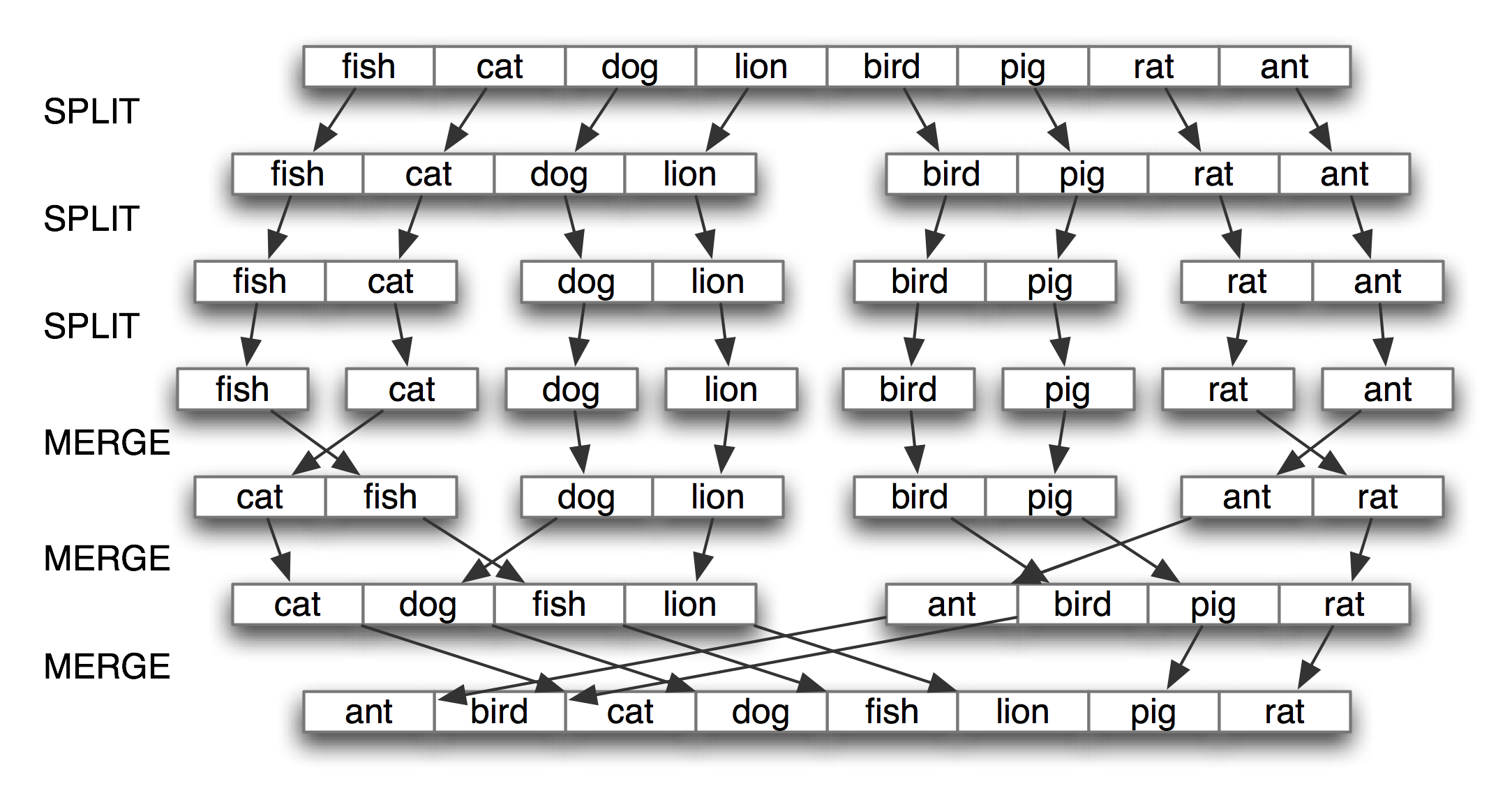### 递归

```>>> def factorial1(n):
...     result = 1
...     for i in range(n):
...         result *= (i+1)
...     return result```

```>>> def factorial2(n):
...     if n == 1:
...         return 1
...     else:
...         return n * factorial2(n-1)```

```>>> def size1(s):
...     return 1 + sum(size1(child) for child in s.hyponyms())```

```>>> def size2(s):
...     layer = [s]...     total = 0
...     while layer:
...         total += len(layer)...         layer = [h for c in layer for h in c.hyponyms()]...     return total```

```>>> from nltk.corpus import wordnet as wn
>>> dog = wn.synset('dog.n.01')
>>> size1(dog)
190
>>> size2(dog)
190```

```def insert(trie, key, value):
if key:
first, rest = key, key[1:]
if first not in trie:
trie[first] = {}
insert(trie[first], rest, value)
else:
trie['value'] = value```

### 权衡空间与时间

```def raw(file):
contents = open(file).read()
contents = re.sub(r'<.*?>', ' ', contents)
contents = re.sub('\s+', ' ', contents)
return contents

def snippet(doc, term):
text = ' '*30 + raw(doc) + ' '*30
pos = text.index(term)
return text[pos-30:pos+30]

print("Building Index...")
files = nltk.corpus.movie_reviews.abspaths()
idx = nltk.Index((w, f) for f in files for w in raw(f).split())

query = ''
while query != "quit":
query = input("query> ")     # use raw_input() in Python 2
if query in idx:
for doc in idx[query]:
print(snippet(doc, query))
else:
print("Not found")```

```def preprocess(tagged_corpus):
words = set()
tags = set()
for sent in tagged_corpus:
for word, tag in sent:
words.add(word)
tags.add(tag)
wm = dict((w, i) for (i, w) in enumerate(words))
tm = dict((t, i) for (i, t) in enumerate(tags))
return [[(wm[w], tm[t]) for (w, t) in sent] for sent in tagged_corpus]```

```>>> from timeit import Timer
>>> vocab_size = 100000
>>> setup_list = "import random; vocab = range(%d)" % vocab_size>>> setup_set = "import random; vocab = set(range(%d))" % vocab_size>>> statement = "random.randint(0, %d) in vocab" % (vocab_size * 2)>>> print(Timer(statement, setup_list).timeit(1000))
2.78092288971
>>> print(Timer(statement, setup_set).timeit(1000))
0.0037260055542```

### 动态规划

Pingala是大约生活在公元前5世纪的印度作家，作品有被称为《Chandas Shastra》的梵文韵律专著。Virahanka大约在公元6 世纪延续了这项工作，研究短音节和长音节组合产生一个长度为n的旋律的组合数。短音节，标记为S，占一个长度单位，而长音节，标记为L，占2个长度单位。例如，Pingala 发现，有5 种方式构造一个长度为4 的旋律：V4 = {LL, SSL, SLS, LSS, SSSS}请看，我们可以将V4分成两个子集，以L开始的子集和以S开始的子集，如(1)所示。

```V4 =
LL, LSS
i.e. L prefixed to each item of V2 = {L, SS}
SSL, SLS, SSSS
i.e. S prefixed to each item of V3 = {SL, LS, SSS}
```

```from numpy import arange
from matplotlib import pyplot

colors = 'rgbcmyk' # red, green, blue, cyan, magenta, yellow, black

def bar_chart(categories, words, counts):
"Plot a bar chart showing counts for each word by category"
ind = arange(len(words))
width = 1 / (len(categories) + 1)
bar_groups = []
for c in range(len(categories)):
bars = pyplot.bar(ind+c*width, counts[categories[c]], width,
color=colors[c % len(colors)])
bar_groups.append(bars)
pyplot.xticks(ind+width, words)
pyplot.legend([b for b in bar_groups], categories, loc='upper left')
pyplot.ylabel('Frequency')
pyplot.title('Frequency of Six Modal Verbs by Genre')
pyplot.show()```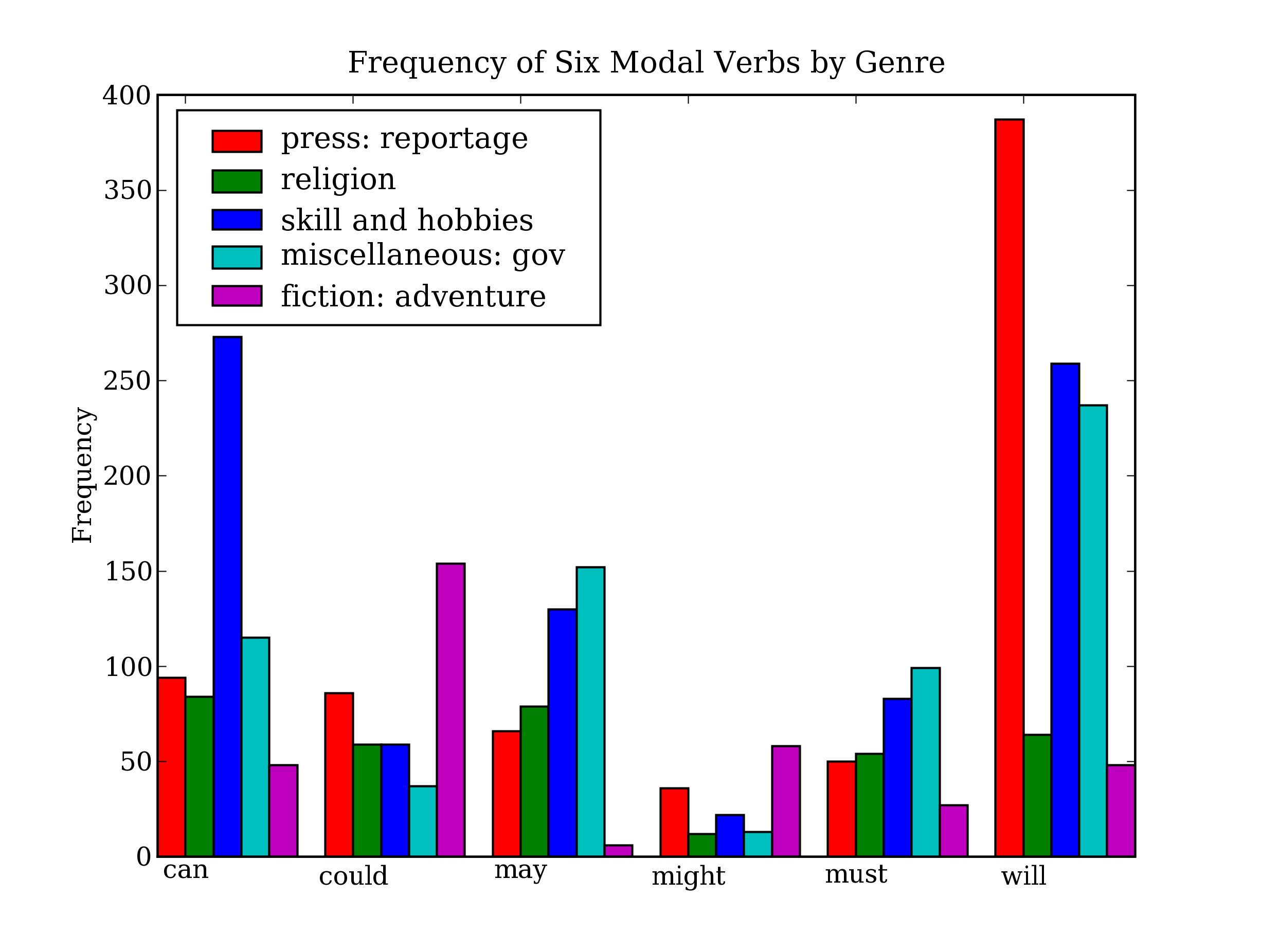```>>> from matplotlib import use, pyplot
>>> use('Agg')>>> pyplot.savefig('modals.png')>>> print('Content-Type: text/html')
>>> print()
>>> print('<html><body>')
>>> print('<img src="modals.png"/>')
>>> print('</body></html>')```

### NetworkX

NetworkX包定义和操作被称为的由节点和边组成的结构。它可以从https://networkx.lanl.gov/得到。NetworkX可以和Matplotlib 结合使用可视化如WordNet的网络结构（语义网络，我们在5介绍过）。4.15中的程序初始化一个空的图，然后遍历WordNet上位词层次为图添加边请注意，遍历是递归的，使用在4.7讨论的编程技术。结果显示在4.16

```import networkx as nx
import matplotlib
from nltk.corpus import wordnet as wn

def traverse(graph, start, node):
graph.depth[node.name] = node.shortest_path_distance(start)
for child in node.hyponyms():
graph.add_edge(node.name, child.name)traverse(graph, start, child)def hyponym_graph(start):
G = nx.Graph()G.depth = {}
traverse(G, start, start)
return G

def graph_draw(graph):
nx.draw_graphviz(graph,
node_size = [16 * graph.degree(n) for n in graph],
node_color = [graph.depth[n] for n in graph],
with_labels = False)
matplotlib.pyplot.show()```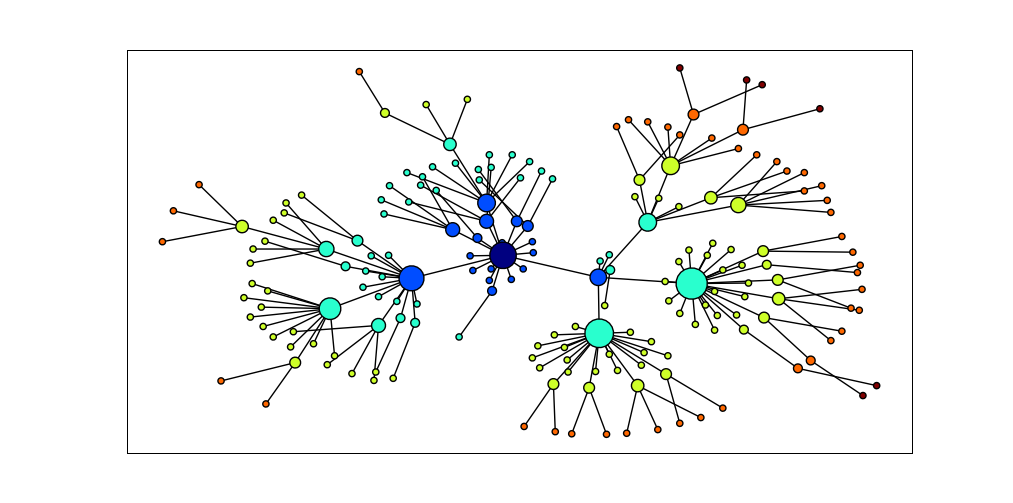### csv

sleep, sli:p, v.i, a condition of body and mind ...
walk, wo:k, v.intr, progress by lifting and setting down each foot ...
wake, weik, intrans, cease to sleep

```>>> import csv
>>> input_file = open("lexicon.csv", "rb")>>> for row in csv.reader(input_file):...     print(row)
['sleep', 'sli:p', 'v.i', 'a condition of body and mind ...']
['walk', 'wo:k', 'v.intr', 'progress by lifting and setting down each foot ...']
['wake', 'weik', 'intrans', 'cease to sleep']```

### NumPy

NumPy包对Python中的数值处理提供了大量的支持。NumPy有一个多维数组对象，它可以很容易初始化和访问：

```>>> from numpy import array
>>> cube = array([ [[0,0,0], [1,1,1], [2,2,2]],
...                [[3,3,3], [4,4,4], [5,5,5]],
...                [[6,6,6], [7,7,7], [8,8,8]] ])
>>> cube[1,1,1]
4
>>> cube.transpose()
array([[6, 7, 8],
[6, 7, 8],
[6, 7, 8]])
>>> cube[2,1:]
array([[7, 7, 7],
[8, 8, 8]])```

NumPy包括线性代数函数。在这里我们进行矩阵的奇异值分解，潜在语义分析中使用的操作，它能帮助识别一个文档集合中的隐含概念。

```>>> from numpy import linalg
>>> a=array([[4,0], [3,-5]])
>>> u,s,vt = linalg.svd(a)
>>> u
array([[-0.4472136 , -0.89442719],
[-0.89442719,  0.4472136 ]])
>>> s
array([ 6.32455532,  3.16227766])
>>> vt
array([[-0.70710678,  0.70710678],
[-0.70710678, -0.70710678]])```

NLTK中的聚类包nltk.cluster中广泛使用NumPy数组，支持包括k-means聚类、高斯EM聚类、组平均凝聚聚类以及聚类分析图。有关详细信息，请输入help(nltk.cluster)

## 6 小结

• Python 赋值和参数传递使用对象引用，例如如果a是一个列表，我们分配b = a，然后任何a上的操作都将修改b，反之亦然。
• is操作测试是否两个对象是相同的内部对象，而==测试是否两个对象是相等的。两者的区别和词符与词类型的区别相似。
• 字符串、列表和元组是不同类型的序列对象，支持常见的操作如：索引、切片、len()sorted()和使用in的成员测试。
• 声明式的编程风格通常会产生更简洁更可读的代码；手动递增循环变量通常是不必要的；枚举一个序列，使用enumerate()
• 函数是一个重要的编程抽象，需要理解的关键概念有：参数传递、变量的作用域和文档字符串。
• 函数作为一个命名空间：函数内部定义的名称在该函数外不可见，除非这些名称被宣布为是全局的。
• 模块允许将材料与本地的文件逻辑的关联起来。一个模块作为一个命名空间：在一个模块中定义的名称——如变量和函数——在其他模块中不可见，除非这些名称被导入。
• 动态规划是一种在NLP 中广泛使用的算法设计技术，它存储以前的计算结果，以避免不必要的重复计算。

## 4.10 深入阅读

Python网站提供大量文档。理解内置的函数和标准类型是很重要的，在http://docs.python.org/library/functions.htmlhttp://docs.python.org/library/stdtypes.html处有描述。我们已经学习了生成器以及它们对提高效率的重要性；关于迭代器的信息，一个密切相关的话题请看http://docs.python.org/library/itertools.html查询你喜欢的Python书籍中这些主题的更多信息。使用Python进行多媒体处理包括声音文件的一个优秀的资源是(Guzdial, 2005)

## 4.11 练习

1. ☼ 使用Python的帮助功能，找出更多关于序列对象的内容。在解释器中输入help(str)help(list)help(tuple)这会给你一个每种类型支持的函数的完整列表。一些函数名字有些特殊，两侧有下划线；正如帮助文档显示的那样，每个这样的函数对应于一些较为熟悉的东西。例如x.__getitem__(y)仅仅是以长篇大论的方式使用x[y]

2. ☼ 确定三个同时在元组和链表上都可以执行的操作。确定三个不能在元组上执行的列表操作。命名一段使用列表替代元组会产生一个Python错误的代码。

3. ☼ 找出如何创建一个由单个项目组成的元组。至少有两种方法可以做到这一点。

4. ☼ 创建一个列表words = ['is', 'NLP', 'fun', '?']使用一系列赋值语句（如words = words）和临时变量tmp将这个列表转换为列表['NLP', 'is', 'fun', '!']现在，使用元组赋值做相同的转换。

5. ☼ 通过输入help(cmp)阅读关于内置的比较函数cmp的内容。它与比较运算符在行为上有何不同？

6. ☼ 创建一个n-grams的滑动窗口的方法在下面两种极端情况下是否正确：n = 1 和n = len(sent)

7. ☼ 我们指出当空字符串和空链表出现在if从句的条件部分时，它们的判断结果是False在这种情况下，它们被说成出现在一个布尔上下文中。实验各种不同的布尔上下文中的非布尔表达式，看它们是否被判断为TrueFalse

8. ☼ 使用不等号比较字符串，如'Monty' < 'Python'当你做'Z' < 'a'时会发生什么？尝试具有共同前缀的字符串对，如'Monty' < 'Montague'阅读有关“字典排序”的内容以便了解这里发生了什么事。尝试比较结构化对象，如('Monty', 1) < ('Monty', 2)这与预期一样吗？

9. ☼ 写代码删除字符串开头和结尾处的空白，并规范化词之间的空格为一个单独的空格字符。

1. 使用split()join()做这个任务
2. 使用正则表达式替换做这个任务
10. ☼ 写一个程序按长度对词排序。定义一个辅助函数cmp_len，它在词长上使用cmp比较函数。

11. ◑ 创建一个词列表并将其存储在变量sent1现在赋值sent2 = sent1修改sent1中的一个项目，验证sent2改变了。

1. 现在尝试同样的练习，但使用sent2 = sent1[:]赋值。再次修改sent1看看sent2会发生什么。解释。
2. 现在定义text1为一个字符串列表的列表（例如表示由多个句子组成的文本）。现在赋值text2 = text1[:]，分配一个新值给其中一个词，例如text1 = 'Monty'检查这对text2做了什么。解释。
3. 导入Python的deepcopy()函数（即from copy import deepcopy），查询其文档，使用它生成任一对象的新副本。
12. ◑ 使用列表乘法初始化n-by-m的空字符串列表的咧表，例如word_table = [[''] * n] * m。当你设置其中一个值时会发生什么事，例如word_table = "hello"解释为什么会出现这种情况。现在写一个表达式，使用range()构造一个列表，表明它没有这个问题。

13. ◑ 写代码初始化一个称为word_vowels的二维数组的集合，处理一个词列表，添加每个词到word_vowels[l][v]，其中l是词的长度，v是它包含的元音的数量。

14. ◑ 写一个函数novel10(text)输出所有在一个文本最后10%出现而之前没有遇到过的词。

15. ◑ 写一个程序将一个句子表示成一个单独的字符串，分割和计数其中的词。让它输出每一个词和词的频率，每行一个，按字母顺序排列。

16. ◑ 阅读有关Gematria的内容，它是一种方法，分配一个数字给词汇，具有相同数字的词之间映射以发现文本隐藏的含义（http://en.wikipedia.org/wiki/Gematria, http://essenes.net/gemcal.htm）。

1. 写一个函数gematria()，根据letter_vals中的字母值，累加一个词的字母的数值：

```>>> letter_vals = {'a':1, 'b':2, 'c':3, 'd':4, 'e':5, 'f':80, 'g':3, 'h':8,
... 'i':10, 'j':10, 'k':20, 'l':30, 'm':40, 'n':50, 'o':70, 'p':80, 'q':100,
... 'r':200, 's':300, 't':400, 'u':6, 'v':6, 'w':800, 'x':60, 'y':10, 'z':7}```
2. 处理一个语料库（nltk.corpus.state_union）对每个文档计数它有多少词的字母数值为666。

3. 写一个函数decode()来处理文本，随机替换词汇为它们的Gematria等值的词，以发现文本的“隐藏的含义”。

17. ◑ 写一个函数shorten(text, n)处理文本，省略文本中前n个最频繁出现的词。它的可读性会如何？

18. ◑ 写代码输出词汇的索引，允许别人根据其含义查找词汇（或它们的发言；词汇条目中包含的任何属性）。

19. ◑ 写一个列表推导排序WordNet中与给定同义词集接近的同义词集的列表。例如，给定同义词集minke_whale.n.01, orca.n.01, novel.n.01tortoise.n.01，按照它们与right_whale.n.01shortest_path_distance()对它们进行排序。

20. ◑ 写一个函数处理一个词列表（含重复项），返回一个按照频率递减排序的词列表（没有重复项）。例如如果输入列表中包含词table的10个实例，chair的9个实例，那么在输出列表中table会出现在chair前面。

21. ◑ 写一个函数以一个文本和一个词汇表作为它的参数，返回在文本中出现但不在词汇表中的一组词。两个参数都可以表示为字符串列表。你能使用set.difference()在一行代码中做这些吗？

22. ◑ 从Python标准库的operator模块导入itemgetter()函数（即from operator import itemgetter）。创建一个包含几个词的列表words现在尝试调用：sorted(words, key=itemgetter(1))sorted(words, key=itemgetter(-1))解释itemgetter()正在做什么。

23. ◑ 写一个递归函数lookup(trie, key)在查找树中查找一个关键字，返回找到的值。扩展这个函数，当一个词由其前缀唯一确定时返回这个词（例如vanguard是以vang-开头的唯一的词，所以lookup(trie, 'vang')应该返回与lookup(trie, 'vanguard')相同的内容）。

24. ◑ 阅读关于“关键字联动”的内容（(Scott & Tribble, 2006)的第5章）。从NLTK的莎士比亚语料库中提取关键字，使用NetworkX包，画出关键字联动网络。

25. ◑ 阅读有关字符串编辑距离和Levenshtein 算法的内容。尝试nltk.edit_distance()提供的实现。这用的是动态规划的何种方式？它使用的是自下而上还是自上而下的方法？[另见http://norvig.com/spell-correct.html]

26. ◑ Catalan 数出现在组合数学的许多应用中，包括解析树的计数（6）。该级数可以定义如下：C0 = 1, and Cn+1 = Σ0..n (CiCn-i)。

1. 编写一个递归函数计算第n个Catalan数Cn
2. 现在写另一个函数使用动态规划做这个计算。
3. 使用timeit模块比较当n增加时这些函数的性能。
27. ★ 重现有关著作权鉴定的(Zhao & Zobel, 2007)中的一些结果。

28. ★ 研究性别特异词汇选择，看你是否可以重现一些http://www.clintoneast.com/articles/words.php的结果

29. ★ 写一个递归函数漂亮的按字母顺序排列输出一个查找树，例如:

```chair: 'flesh'
---t: 'cat'
--ic: 'stylish'
---en: 'dog'
```

## Docutils System Messages

System Message: ERROR/3 (ch04.rst2, line 1791); backlink

Unknown target name: "first-run".

System Message: ERROR/3 (ch04.rst2, line 1791); backlink

Unknown target name: "second-run".